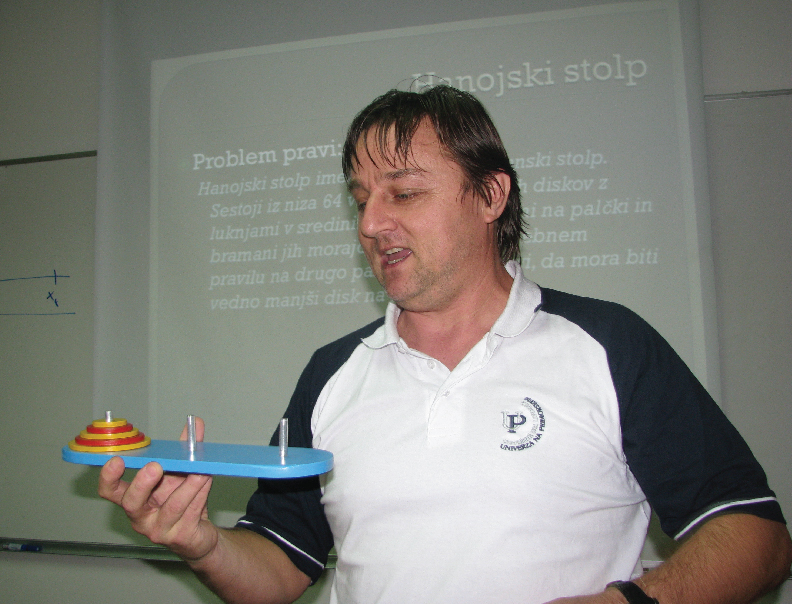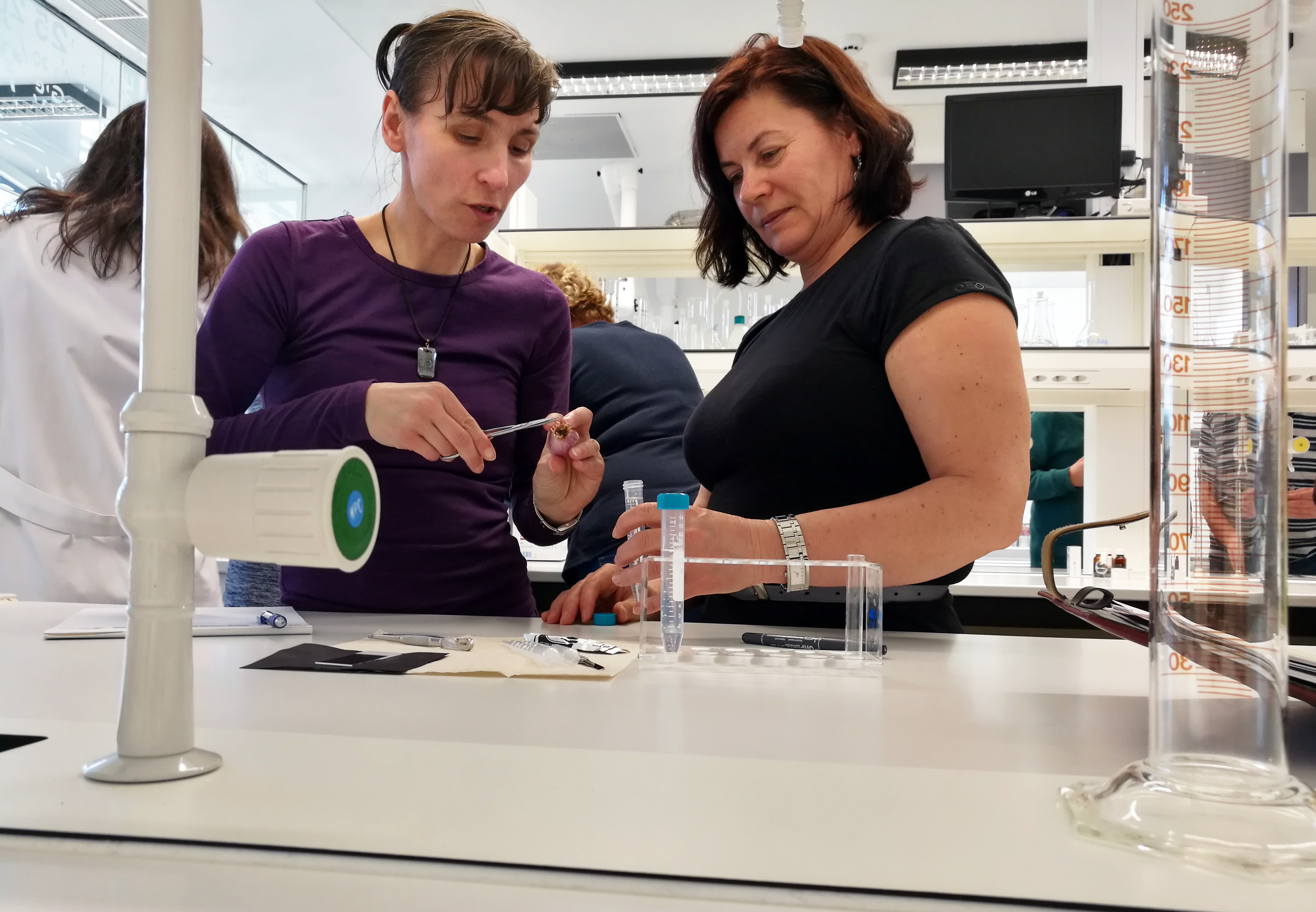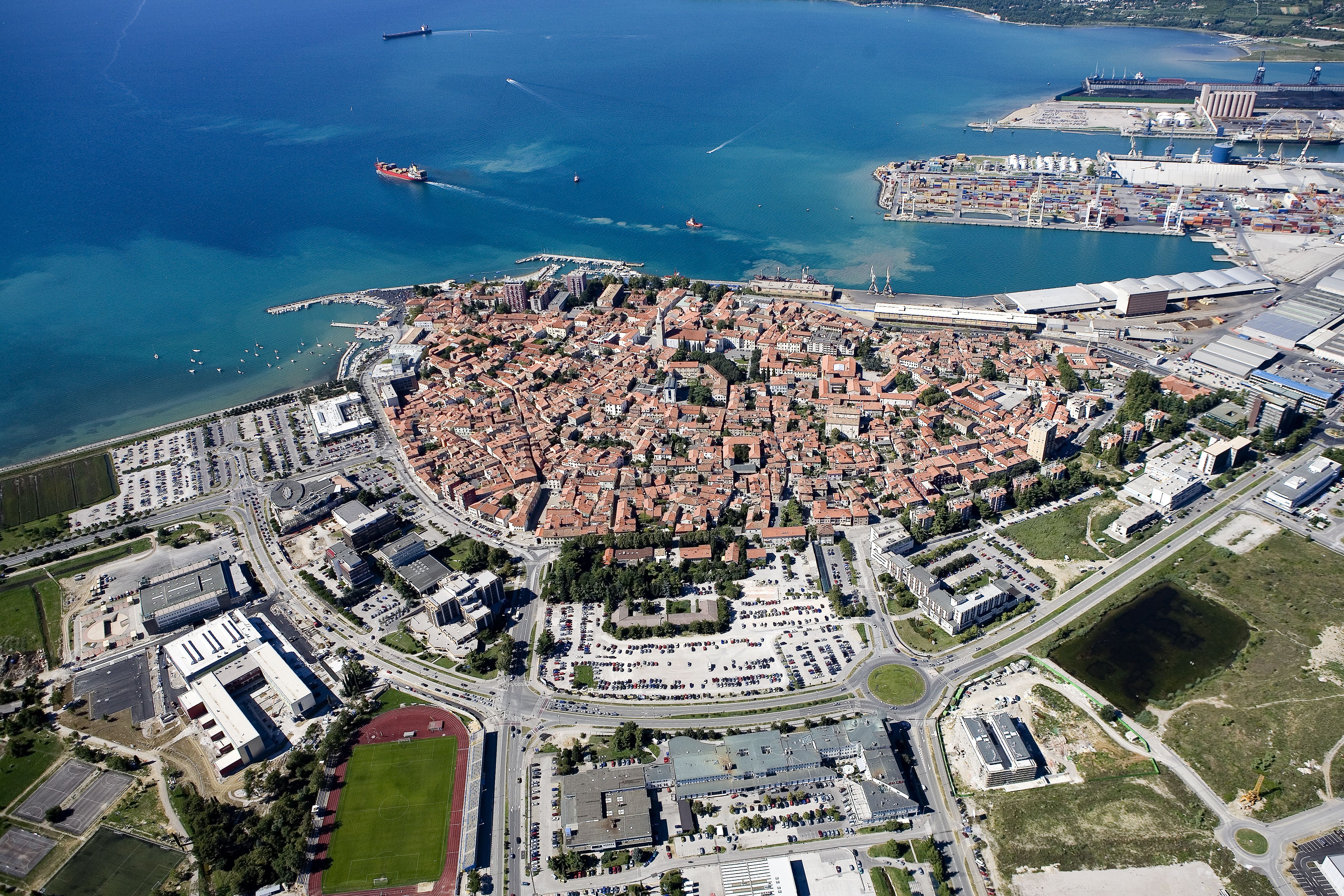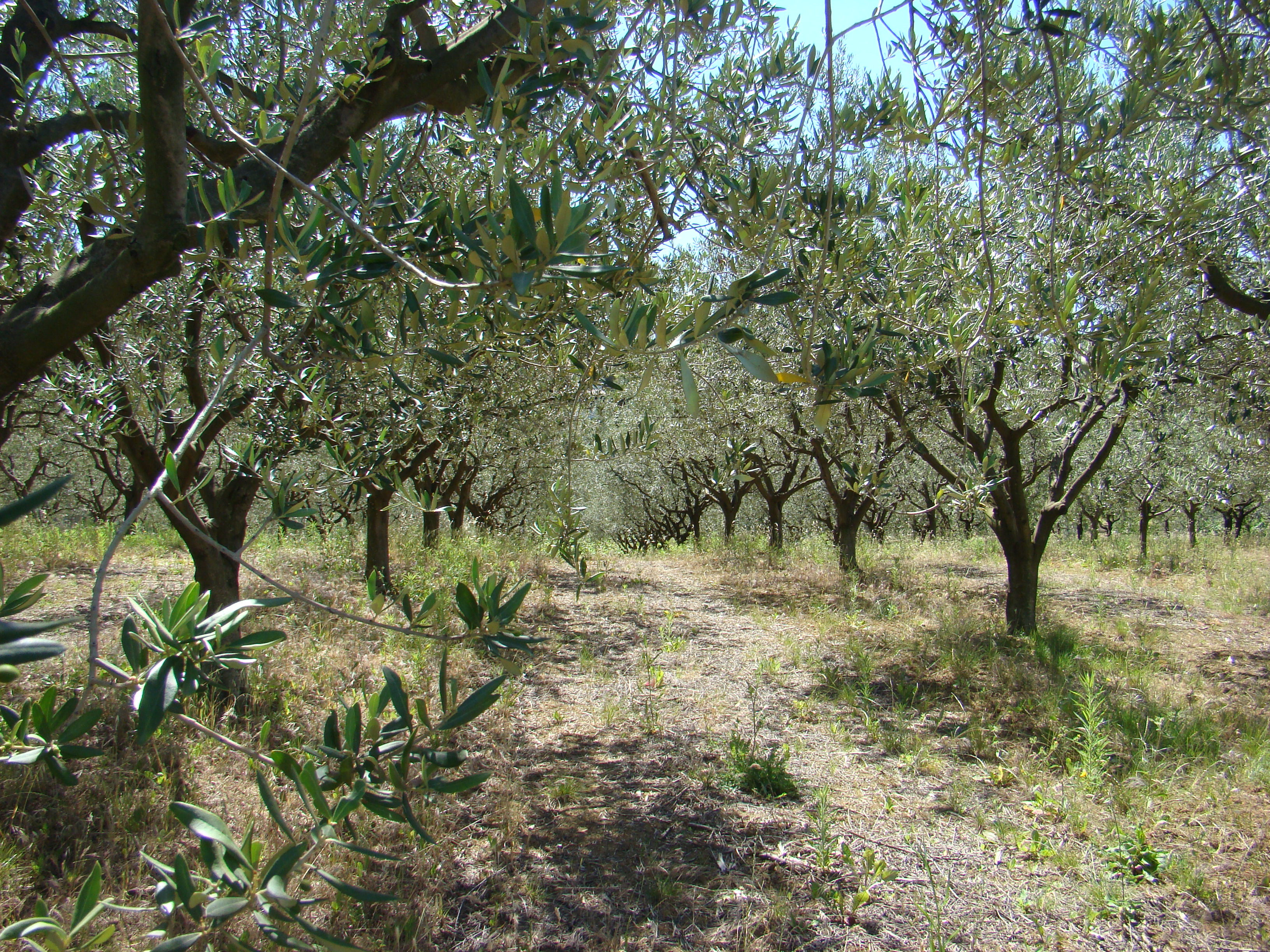Univerza na Primorskem Fakulteta za matematiko, naravoslovje in informacijske tehnologijeSI | EN# Raziskovalni matematični seminar - Arhiv

 2022 2021 2020 2019 2018 2017 2016 2015 2014 2013 2012 2011 2010
 1 2 3 4 5 6 7 8 9 10 11 12
Datum in ura / Date and time: 29.4.13
(10:00-11:00)
Predavalnica / Location: FAMNIT-SEMIN
Predavatelj / Lecturer: Prof. György Kiss (Eötvös Loránd University, Hungary)
Naslov / Title: On m-factorizations of complete multigraphs and designs
Vsebina / Abstract:

The complete multigraph $\lambda K_{v}$ has $v$ vertices and $\lambda$ edges
joining each pair of vertices. An $m$-factor of the complete multigraph
$\lambda K_{v}$ is a set of pairwise vertex-disjoint $m$-regular subgraphs,
such that these subgraphs induce a partition of the vertices. An
$m$-factorization of $\lambda K_{v}$ is a set of pairwise edge-disjoint
$m$-factors such that these $m$-factors induce a partition of the edges. If
the $m$-factors are pairwise distinct, then it is called
\emph{simple}. Furthermore, an $m$-factorization of $\lambda K_{v}$ is
decomposable if there exist positive integers $\lambda_{1}$ and $\lambda_{2}$
such that $\lambda_{1}+\lambda_{2}=\lambda$ and $\lambda K_{v}$ is the union
of the $m$-factorizations $\lambda_{1}K_{v}$ and $\lambda_{2}K_{v}$, otherwise
it is called \emph{indecomposable}.

In this talk the existence of $m$-factorizations of $\lambda K_v$
for different values of $m$, $\lambda$ and $v$ is studied. Some new
infinite families of simple, indecomposable factorizations arising from
finite geometries will be presented.

The talk is based on a joint work with Christian Rubio-Montiel (UNAM, Mexico).

Datum in ura / Date and time: 22.4.13
(10:00-11:00)
Predavalnica / Location: FAMNIT-SEMIN
Predavatelj / Lecturer: Dijana Ilišević (University of Zagreb, Croatia)
Naslov / Title: Generalized Bicircular Projections
Vsebina / Abstract:

A projection P on a complex Banach space X is called a generalized bicircular projection if the mapping
P + a( I-P ) is an isometry for some modulus one complex number a.  The aim of this talk is to describe the structure of these mappings on some matrix and operator spaces.

Datum in ura / Date and time: 15.4.13
(10:00-11:00)
Predavalnica / Location: FAMNIT-SEMIN
Predavatelj / Lecturer: Arjana Žitnik (FMF, Ljubljana)
Naslov / Title: TheCover
Vsebina / Abstract:

Each finite graph on n vertices determines a special (n-1)-fold covering graph that we call TheCover. Several equivalent definitions and basic properties about this remarkable construction are presented. In particular, we show that TheCover of a k-connected graph is k-connected, TheCover of a planar graph is planar and TheCover of a hamiltonian graph is hamiltonian. By studying automorphisms of these covers we also understand the structure of their automorphism groups. A particular nice property is that every automorphism of the base graph lifts to an automorphism of its TheCover. We also show that TheCover of a 3-connected graph X is never a regular cover of X. This is a joint work with Aleksander Malnič and Tomaž Pisanski.

Datum in ura / Date and time: 8.4.13
(10:00-11:00)
Predavalnica / Location: FAMNIT-SEMIN
Predavatelj / Lecturer: doc. dr. Polona Oblak (Fakulteta za računalništvo in informatiko, Univerza v Ljubljani.)
Naslov / Title: On commuting graphs
Vsebina / Abstract:

The \emph{commuting graph} $\Gamma(S)$ of a semigroup (or a semiring) $S$ is the graph, whose vertex set is the set of all noncentral elements of $S$ and $x-y$ is an edge in $\Gamma(S)$ if $xy=yx$ and $x \ne y$. The commuting graphs are an illustrative way of describing centralizers of elements in certain algebraic structures. In the talk, we introduce commuting graphs and give some recent results on diameters of commuting graphs of different algebraic structures. The main part of the talk will be concentrating on commuting graphs of matrices over rings and semirings.